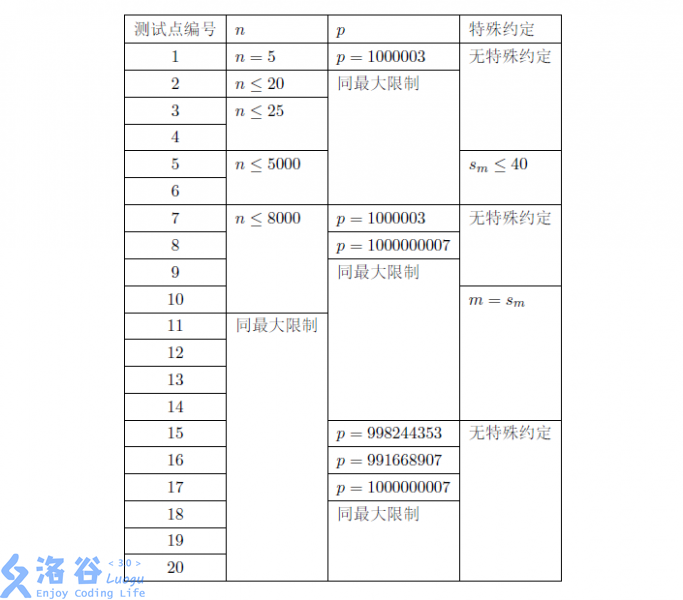# P3784 [SDOI2017]遗忘的集合

• 156通过
• 448提交
• 题目提供者 deluxurous
• 评测方式 云端评测
• 标签 构造 生成函数 莫比乌斯反演 各省省选 2017 山东 O2优化 高性能
• 难度 NOI/NOI+/CTSC
• 时空限制 5000ms / 524MB
• 提示：收藏到任务计划后，可在首页查看。

## 题目描述

小Q在他的个人主页上放出了一个悬赏：征集只含正整数的非空集合$S$ ，其中的每个元素都不超过$n$ ，并且满足一些附加条件。

众所周知，我们可以很轻松地对于任意不超过$n$的正整数$x$，计算出把$x$表示成$S$中元素之和的方案数$f(x)$，在这里我们约定，在任意方案中每个数字可以出现多次，但是不考虑数字出现的顺序。

例如，当$S=\{1,2,3,4,5\}$时，我们可以计算出$f(2) = 2, f(3) = 3, f(4) = 5, f(5) = 7$。

再例如，当$S=\{1,2,5\}$时，我们可以计算出$f(4) = 3, f(5) = 4, f(6) = 5, f(7) = 6$。

麻烦地是现在小Q忘记了$S$里有哪些元素，幸运地是他用存储设备记录下了所有$f(i) \mathrm{\ mod\ } p$的值，小Q希望你能利用这些信息帮他恢复出$S$原来的样子。

具体来说，他希望你找到这样一个正整数的非空集合$S$，其中的每个元素都不超过$n$，并且对于任意的$i = 1, 2,\cdots ,n$，满足把$i$表示成$S$中元素之和的方案数在模$p$意义下等于$f(i)$，其中$p$是记录在存储设备中的一个质数。他向你保证：一定存在这样的集合$S$ 。

然而，小Q觉得他存储的信息并不足以恢复出唯一的$S$，也就是说，可能会存在多个这样的集合$S$，所以小Q希望你能给出所有解中字典序最小的解。

对于满足条件的两个不同的集合$S_1$和$S_2$，我们认为$S_1$的字典序比$S_2$的字典序小，当且仅当存在非负整数$k$，使得$S_1$的前$k$小元素与$S_2$的前$k$小元素完全相等，并且，要么$S_1$的元素个数为$k$，且$S_2$的元素个数至少为$(k + 1)$，要么$S_1$和$S_2$都有至少$(k + 1)$个元素，且$S_1$的第$(k + 1)$小元素比$S_2$的第$(k + 1)$小元素小。

## 输入输出格式

输入格式：

第一行包含两个整数$n$和$p$，满足$p$是质数。

第二行包含$n$个整数$f(1), f(2),\cdots , f(n)$ ，含义见题目描述。

保证每一行中相邻的整数由恰好一个空格隔开，并且末尾没有多余的空格。

输出格式：

你需要输出两行信息来描述字典序最小的解，其中第一行包含一个整数$m (m > 0)$，表示$S$的元素个数，第二行包含$m$个正整数$s_1, s_2,\cdots ,s_m$，表示将$S$的所有元素按升序排序后得到的序列。

你需要保证输出的每一行中相邻的整数由恰好一个空格隔开，并且每一行的末尾没有多余的空格。

## 输入输出样例

输入样例#1： 复制
5 1000003
1 2 3 5 7
输出样例#1： 复制
5
1 2 3 4 5
输入样例#2： 复制
9 1000003
1 2 2 3 4 5 6 7 8
输出样例#2： 复制
3
1 2 5

## 说明

对于$100\%$的数据，有$1 \leq n < 2^{18} , 10^6 \leq p < 2^{30} , 0 \leq f(i) < p (i=1,2, \cdots , n)$。提示
标程仅供做题后或实在无思路时参考。
请自觉、自律地使用该功能并请对自己的学习负责。
如果发现恶意抄袭标程，将按照I类违反进行处理。